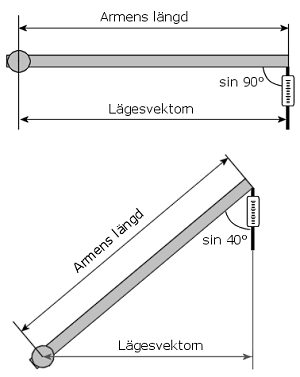Home Forums Webstore Manuals Solar Wind Hydro Electricity & Electronics Heating Calculators Projects Other Links About 24 volt ContactOtherPedal PowerThe VelomobileTorque & PowerGear & Pully typesConversion TablesAWG to mm tableSpeed conversionJoineriesA patio for our cottageSök på 24volt.eu

 Web 24volt.eu

Besökare/Visitors
online:
1

# Torque and Power

The torque (or moment of force) may be calculated using the following formula:

τ = F x r x sinΦ

sinΦ equals 1 if the angle is 90°. If the arm with which the torque is measured is horizontal, the angle may therefore be disregarded. The position vector r therefore equals the length of the arm, between the anchor point of the scales and the centre of the turning axis. The formula may then be written as:

τ = F x r

r (the position vector) is measured in metres and since F = m x g, where m stands for mass in kg and g for earths gravity (9.81), you can simplify things greatly for yourself by making the arm 1 metre long. The torque measured in Newton metres (Nm) then equals about 10 times what the scales are showing. Thus, each kilogram will equal 10 Nm.

In order to estimate the torque, use the calculator below. Please note that the arm should be weighed for correct measuring results. Lift the arm to a point straight above the stationary scales, using another pair of scales until it is in a horizontal position. Read the weight and add it to the mass column in the calculator below.

N.B: Use a full stop when using decimals, not a comma!
 Position vector (r): metres Angle (sinΦ): ° Mass: kgResult:
 Torque: Nm
 Once you are familiar with the torque at different revolutions per minute (rpms) the power is easy to calculate. The formula for power calculation is: P = τ x n x 2 x pi τ = Torque n = roations/ second pi = 3,14
 Torque (τ): Nm Revs: rpmResultat:
 Power: WattsIn the picture above the spring weighing scale is hidden behind the rock. When the screws are tightened around the axis of the wheel, the wheel slows and the scales may be read. Then the screws are tightened again followed by a new reading. Repeat and note the rpm of the wheel and the weight on the scales until the wheel is completely still.When you have collected all the data you need for torque and effect, it is possible to draw a curve diagram showing effect and torque.